# 一、前言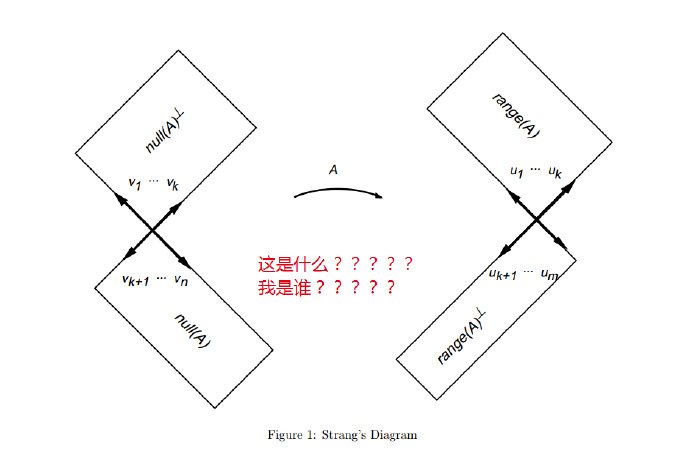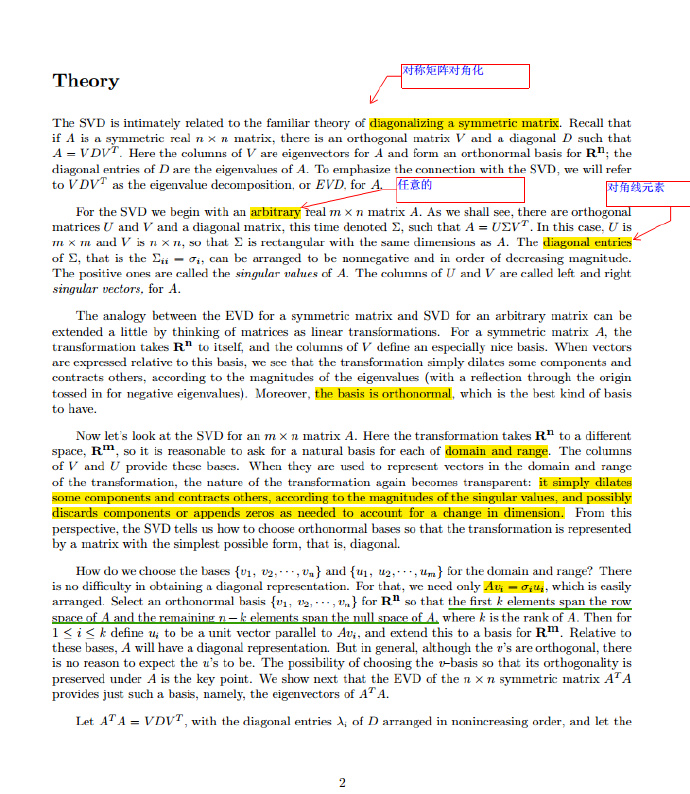# 二、奇异值分解：从特征值分解的角度

\begin{equation}\begin{split}
A=U \Sigma V^{T}
\label{1}
\end{split}\end{equation}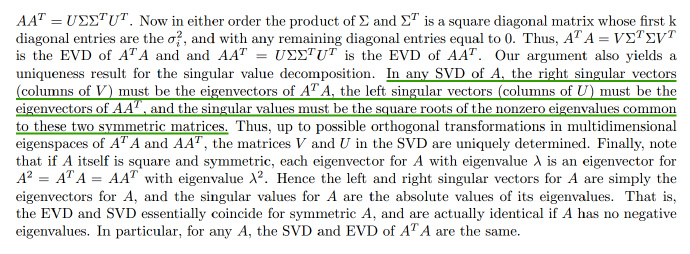$A$的左右奇异向量如果用列向量的形式表示

$\Sigma$的对角元素为$\sigma_i$，那么$A$可以分解为一系列的线性组合

\begin{equation}\begin{split}
A = \sigma_1u_1v_1^{T} + \sigma_2u_2v_2^{T}+···+\sigma_nu_nv_n^{T} \
\label{svd}
\end{split}\end{equation}

# 三、用 Python 实现 SVD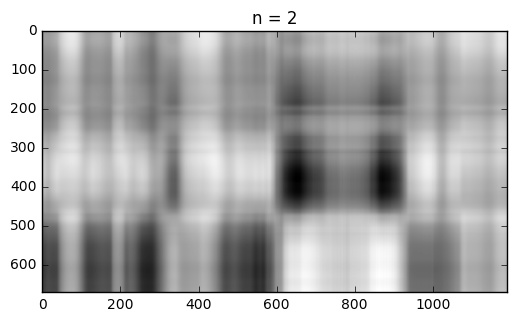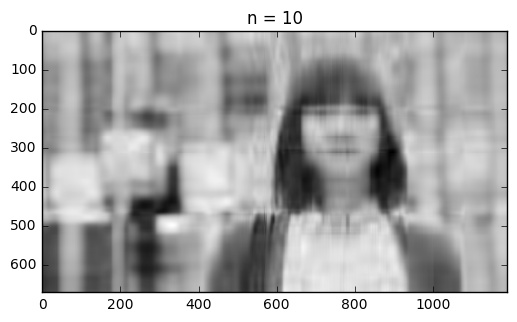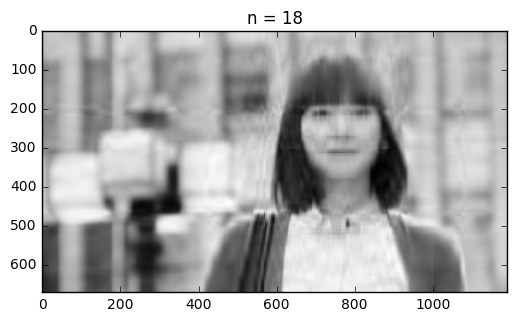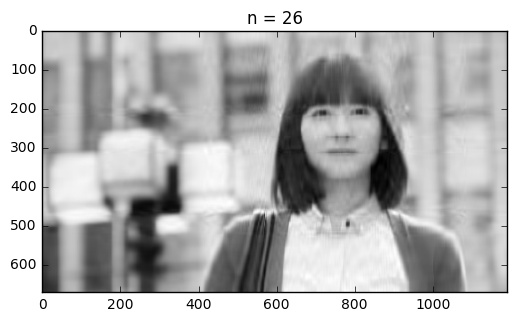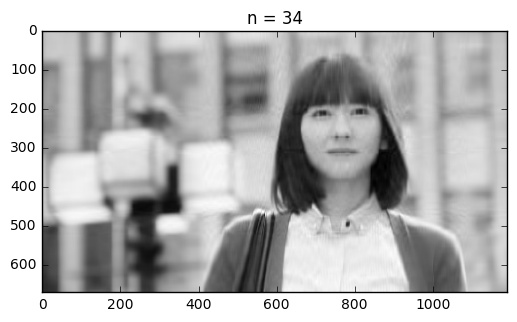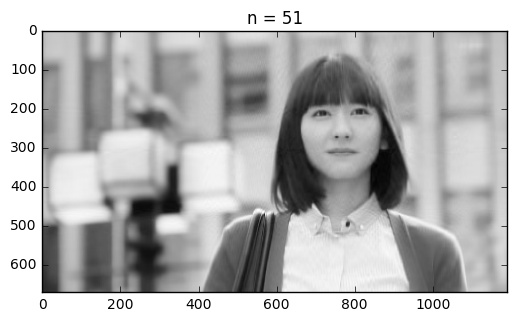# 参考链接

------本文结束，欢迎收藏本站、分享、评论或联系作者！------

(>看完记得五星好评哦亲<)
0%